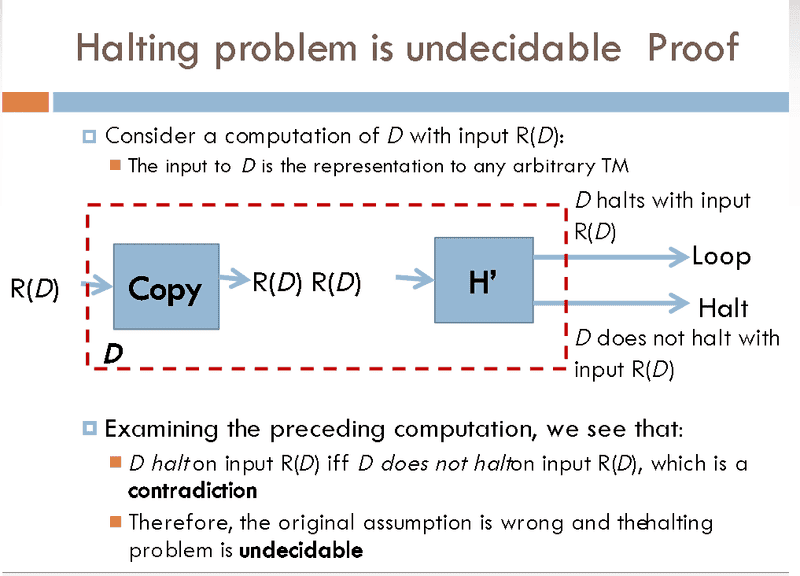Halting problem is undecidable proof confusion-:

shivajikobardan

New member
THE HALTING PROBLEM - PROOF. Review  What makes a problem decidable?  3 properties of an efficient algorithm?  What is the meaning of “complete”, “mechanistic”, - ppt download
This is the context I am talking about.What contradiction occur here? We begin by telling that there is a Turing machine H that solves the halting problem. So how does this contradicts? Can you tell me about that?

What contradiction occur here? We begin by telling that there is a Turing machine H that solves the halting problem. So how does this contradicts? Can you tell me about that?